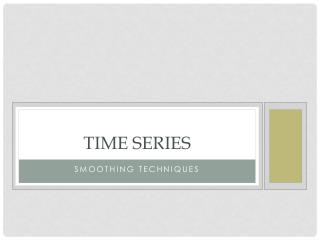DownloadDownload PresentationTime Series

# Time Series

Télécharger la présentation## Time Series

- - - - - - - - - - - - - - - - - - - - - - - - - - - E N D - - - - - - - - - - - - - - - - - - - - - - - - - - -
##### Presentation Transcript

1. Time Series Smoothing techniques

2. Components of a time series Components of a time series • Seasonal effect • Long term trend • Cyclical effect • Irregularity, random variation or random error

3. Seasonal effect

4. Seasonal effect Seasonal variation • Regular period usually based on calendar. • Could be literally seasonal but may be monthly, daily, does not have to related to the seasons (summer, autumn etc)

5. Long term trend

6. Long term trend Long term trend • The most, slowly changing component • The long term component, either upward or downward direction or stationary. • Rate of change • Due to long term changes such as population growth or change, technological change

7. Random/ Irregular component

8. Random/ Irregular component Random/ Irregular component • Most rapidly changing component • Totally unpredictable • What is left over after other components have been taken out • Look for ramps, spikes, troughs etc.

9. Cyclical effect

10. Cyclical effect Cyclical variation • The next most slowly changing component • Recurrent, wave-like changes in the series • The period and amplitude of the wave are not predictable • Often due to the business cycle, prosperity, recession, depression and recovery.

11. Smoothing Techniques • Often it is hard to see the trend of data because of random fluctuations. Getting rid of these bumps or noise helps to “smooth” the data, summarise it and reveal the long term trend. One way to do this is to work out the Moving Means • Moving Means are easy to calculate. All data contributes to the mean, so an extreme piece of data can causes the moving mean to fluctuate dramatically. • Choosing the order - The most effective approach in smoothing raw data by using moving averages method, is making the order the same number as there are intervals within the seasonal cycle for the data (e.g. monthly – order 12, seasons – order 4, daily - order 7, weekdays – order 5 ).

12. Trend After we have smoothed the data, a better approximation of the overall trend will be given by the graph. If the smoothed graph is a horizontal line then the overall trend is to stay constant/stationary.

13. Trend If the smoothed graph is best approximated by a straight line then the trend is a constant addition (or subtraction) each complete cycle. That is, the change (e.g. profit or loss) is consistent each cycle. • If the smoothed graph is best approximated by a curve then the trend is a multiplier (e.g. inflation)

14. Trend If the smoothed graph is best approximated by a curve then the trend is a multiplier (e.g. inflation) The two types of model • The additive model is suitable where the periodic variation is of constant magnitude • The multiplicative model is suitable where the periodic variation is of increasing magnitude

15. Calculating Seasonal Effects INDIVIDUAL SEASONAL EFFECT = RAW VALUE – MOVING MEAN It gives a value to how much the raw data is above or below the smoothed data value so some seasonal effects will be negative.

16. Calculating average seasonal effect

17. Seasonally Adjusting Data If we want to remove the seasonality of the data that we are measuring then we need to calculate the seasonally adjusted data. Seasonally adjusted values (SAV) are raw data values with the seasonal effect removed, i.e. SAV = RAW – ASE